$r_{wg}$是用于评估评分者内部一致性(inter-rater agreement index)的指标，

Intraclass相关系数（ICC）：这是一种用于评估评分者内部一致性的常用指标，可以考虑到评分者与评分者之间的关系。

### 有哪些统计指标可以计算评分者内部一致性

• Kappa系数：这是一种常用的评分者内部一致性指标，它考虑了随机误差，并且可以应用于多个评分者和多个评分类别。
• Pearson相关系数：这是一种常用的相关系数，可以度量评分者间的线性关系。
• 均方根误差：这是一种常用的评分者内部一致性指标，可以表示评分者对于同一评分标准的评分结果的离散程度。
• Fleiss’ Kappa系数：这是一种用于多评分者和多评分类别情况的评分者内部一致性指标。
• Intraclass相关系数（ICC）：这是一种用于评估评分者内部一致性的常用指标，可以考虑到评分者与评分者之间的关系。
• James’ $r_{wg}$

### $r_{wg}$ 计算公式

$$r_{wg} = 1 - (S^2/t_E^2)$$

• $S^2$代表方差
• $t_E^2$代表期望方差（评分者完全随机情况下的方差）

$t_E^2$的计算公式如下(假定均匀分布)：

$$t_E^2 = (A^2 - 1) / 12$$

• A代表评分等级，比如5级计分， A=5

$S^2$趋近于0时，评分者的评分完全一致， $r_{wg}$接近于1；

### ICC 组内相关系数 计算公式

Intraclass Correlation Coefficient (ICC) 公式可以表示如下：

ICC = (MSB - MSW) / (MSB + (k-1) * MSW)

MSB：组间均方 (between-group mean square）
MSW：组内均方( within-group mean square）
k：表示评分者数量

• 对于每个评分者，计算该评分者给出的所有评分的平均值。
• 对所有评分者的平均评分求平均值，得到所有评分的总平均值。
• 对每个评分者的平均评分，计算该评分与所有评分的总平均值的差的平方。
• 将每个评分者的差的平方相加，得到MSB。
• 对于每个评分，计算该评分与该评分者的平均评分的差的平方。
• 将每个评分者的差的平方相加，除以评分数量，得到MSW。
• 用MSB和MSW计算ICC，公式如上。

### 使用Excel计算评分者一致性$r_{wg}$

#### 数据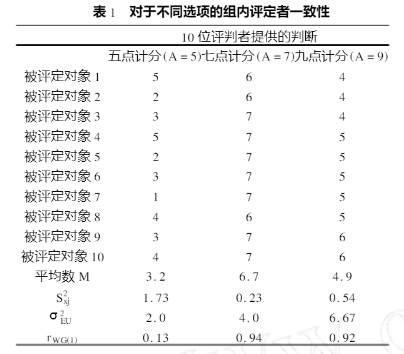#### 录入数据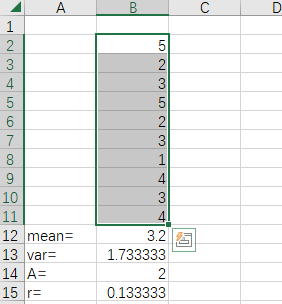#### 计算方差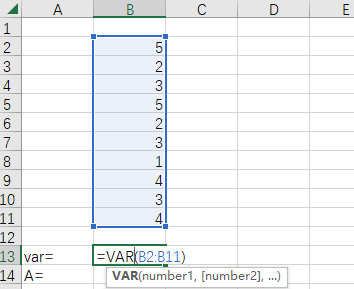#### 计算理想方差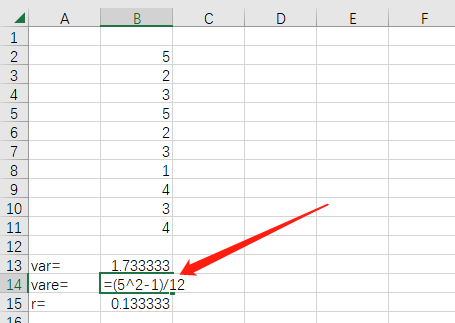#### 计算$r_{wg}$### 使用Excel计算组内相关系数ICC

#### 数据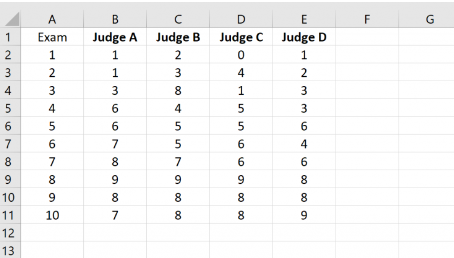#### ANOVA分析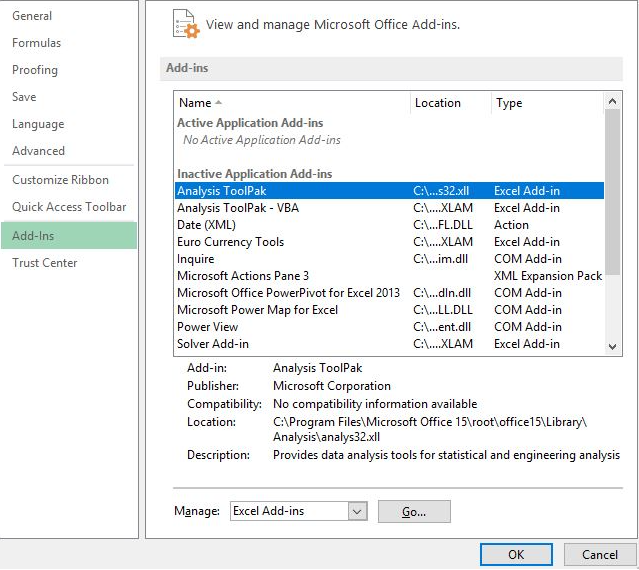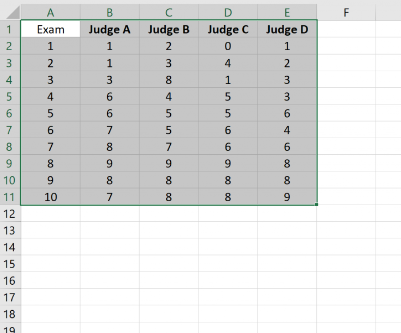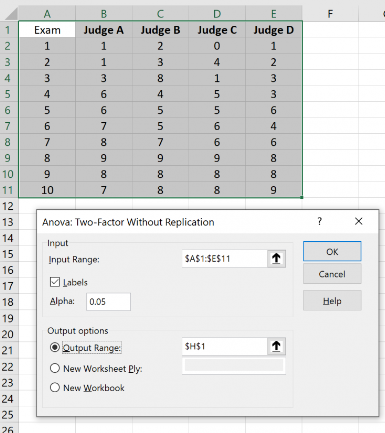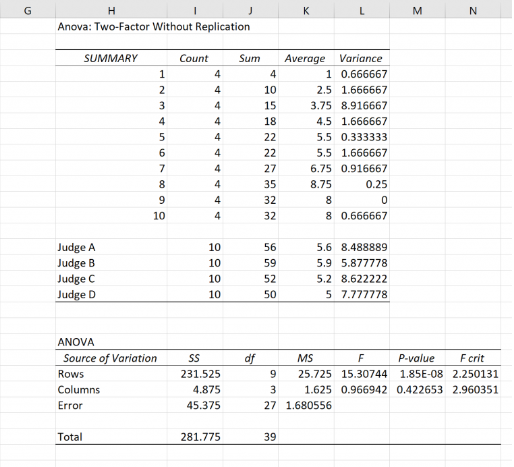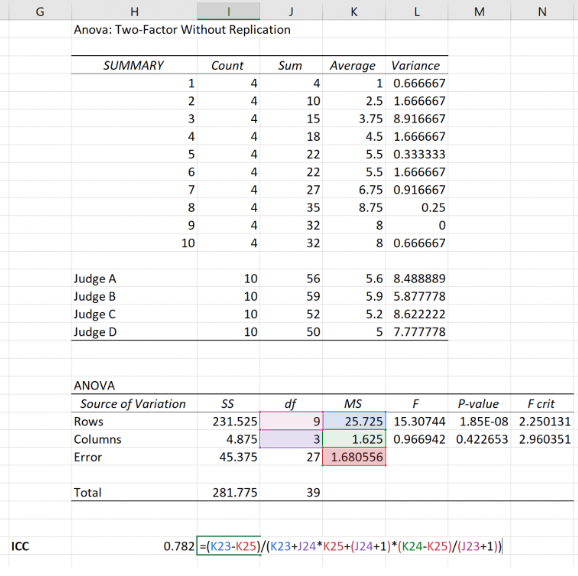#### 解读公式

• MSRow = 25.7
• MSCol = 1.6
• MSE = 1.68

$$ICC = {var(\beta) \over {var(\alpha) + var(\beta) + var(e)}}$$

var(β) = (MSRow – MSE)/k = (25.7 – 1.68)/4

var(ε) = MSE = 1.68

var(α) = (MSCol – MSE)/n = (1.6– 1.68)/10

### 赞助